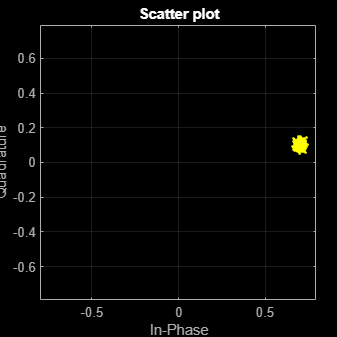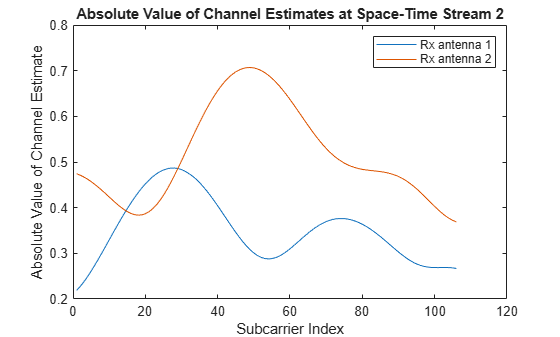# wlanEHTLTFChannelEstimate

Channel estimation using EHT-LTF

Since R2023a

## Syntax

``chEst = wlanEHTLTFChannelEstimate(demodSig,cfg)``
``chEst = wlanEHTLTFChannelEstimate(demodSig,cfg,ruNumber)``
``[chEst,chEstPilots] = wlanEHTLTFChannelEstimate(___)``
``````[chEst,chEstPilots] = wlanEHTLTFChannelEstimate(___,FrequencySmoothingSpan=span)``````

## Description

example

````chEst = wlanEHTLTFChannelEstimate(demodSig,cfg)` returns the channel estimate at the extremely high-throughput long training field (EHT-LTF) using the demodulated EHT-LTF signal, `demodSig`, given the parameters specified in the configuration object `cfg`. For more information about this field, see EHT-LTF.```

example

````chEst = wlanEHTLTFChannelEstimate(demodSig,cfg,ruNumber)` returns the channel estimate for the resource unit (RU) or multiple resource unit (MRU) of interest. This input is required when the PPDU type is OFDMA.```

example

````[chEst,chEstPilots] = wlanEHTLTFChannelEstimate(___)` also returns the channel estimate at each pilot subcarrier location for each demodulated EHT-LTF OFDM symbol, `chEstPilots`, for any input argument combination from the previous syntaxes.```

example

``````[chEst,chEstPilots] = wlanEHTLTFChannelEstimate(___,FrequencySmoothingSpan=span)``` specifies frequency smoothing using a name-value argument in addition to any input argument combination from the previous syntaxes.```

## Examples

collapse all

Calculate and plot the channel estimate of a non-OFDMA EHT MU transmission using the EHT long training field.

Create a non-OFDMA EHT MU configuration object with a channel bandwidth of 320 MHz. Generate a time-domain waveform for the configuration.

```chanBW = "CBW320"; cfg = wlanEHTMUConfig(chanBW); txSig = wlanWaveformGenerator([1;0;0;1],cfg);```

Multiply the transmitted signal by 0.7 + 0.1i and pass it through an AWGN channel with a signal-to-noise ratio of 30 dB.

`rxSig = awgn((0.7 + 0.1i)*txSig,30);`

Extract the EHT-LTF field indices and demodulate the EHT-LTF. Perform channel estimation, specifying a frequency smoothing span of `3`.

```indEHTLTF = wlanFieldIndices(cfg,"EHT-LTF"); demodSig = wlanEHTDemodulate(rxSig(indEHTLTF(1):indEHTLTF(2),:),"EHT-LTF",cfg); chEst = wlanEHTLTFChannelEstimate(demodSig,cfg,FrequencySmoothingSpan=3);```

Plot the channel estimate.

```scatterplot(chEst) grid```Calculate and plot the channel estimate of an EHT MU format MIMO channel by using the long training field.

Create an OFDMA EHT MU configuration object. Set the allocation index to `25`. This setting specifies a configuration with three users. One user has a 26-tone RU and the remaining two each have a 106-tone RU. Specify two transmit antennas, and two space-time streams for each user. Specify the RU number of interest.

```allocationIndex = 25; cfg = wlanEHTMUConfig(allocationIndex,NumTransmitAntennas=2); for i = 1:3 cfg.User{i}.NumSpaceTimeStreams = 2; end ruNumber = 3;```

Generate a time-domain waveform for the configuration.

`txSig = wlanWaveformGenerator([1;0;0;1],cfg);`

Create a TGax channel System object™ with a channel bandwidth of 20 MHz. Pass the transmitted signal through the TGax MIMO channel.

```tgax = wlanTGaxChannel; tgax.ChannelBandwidth = "CBW20"; tgax.NumTransmitAntennas = 2; tgax.NumReceiveAntennas = 2; rxSig = tgax(txSig);```

Extract the EHT-LTF field indices and demodulate the EHT-LTF for the RU of interest. Perform channel estimation at each pilot subcarrier location.

```indEHTLTF = wlanFieldIndices(cfg,"EHT-LTF"); demodSig = wlanEHTDemodulate(rxSig(indEHTLTF(1):indEHTLTF(2),:),"EHT-LTF",cfg,ruNumber); [est,estPilots] = wlanEHTLTFChannelEstimate(demodSig,cfg,ruNumber);```

Plot the absolute value of the channel estimate at all occupied subcarriers for the second of the two space-time streams at both receive antennas.

```plot(abs(est(:,2,1))) hold on plot(abs(est(:,2,2))) xlabel("Subcarrier Index") title("Absolute Value of Channel Estimates at Space-Time Stream 2") ylabel("Absolute Value of Channel Estimate") legend("Rx antenna 1","Rx antenna 2")```## Input Arguments

collapse all

Demodulated EHT-LTF signal, specified as an NST-by-NSYM-by-NR array. NST is the number of occupied subcarriers, NSYM is the number of demodulated EHT-LTF OFDM symbols, and NR is the number of receive antennas.

Data Types: `single` | `double`
Complex Number Support: Yes

Physical layer (PHY) format configuration, specified as an object of type `wlanEHTMUConfig`, `wlanEHTTBConfig`, or `wlanEHTRecoveryConfig`.

Number of the RU or MRU of interest, specified as a positive integer. This input specifies the location of the RU or MRU in the channel. For example, consider a 20 MHz transmission with one 106+26-tone RU, one 52+26-tone MRU, and one 26-tone RU, in order of absolute frequency. For this allocation:

• RU 1 corresponds to the 106+26-tone MRU at the lowest absolute frequency (size 106+26, index 1).

• RU 2 corresponds to the 52+26-tone MRU at the next lowest absolute frequency (size 52+26, index 2).

• RU 3 corresponds to the 26-tone RU at the highest absolute frequency (size 26, index 3).

Note

• For an OFDMA-type EHT MU PPDU, this input is required.

• For a non-OFDMA-type EHT MU PPDU, this input is not required.

• For an EHT TB PPDU, this input is not required.

• This input is not required when you specify `cfg` as a `wlanEHTRecoveryConfig` object.

Data Types: `single` | `double`

Span of the frequency smoothing filter, specified as a positive odd integer. The span is expressed as a number of subcarriers. The function applies frequency smoothing when `span` is greater than one. For more information on when to specify this input, see Frequency Smoothing.

## Output Arguments

collapse all

Channel estimate between all combinations of space-time streams and receive antennas, returned as an NST-by-NSTS,total-by-NR array. NST is the number of occupied subcarriers. NSTS,total is the total number of space-time streams for all users. In the single-user case, NSTS,total=NSTS. In the multi-user case, NSTS,total is the sum of the values of NSTS for each user of the RU of interest. NR is the number of receive antennas.

Data Types: `single` | `double`
Complex Number Support: Yes

Channel estimate at each pilot subcarrier location for each EHT-LTF symbol, returned as an NSP-by-NSYM-by-NR array. NSP is the number of pilot subcarriers, NSYM is the number of demodulated EHT-LTF OFDM symbols, and NR is the number of receive antennas. The function performs this estimate assuming one space-time stream at the transmitter.

Data Types: `single` | `double`
Complex Number Support: Yes

collapse all

### EHT-LTF

The EHT-LTF is located between the EHT-STF and data field of an EHT packet. As described in Section 36.3.12.10 of IEEE® P802.11be™/D3.0 , the receiver can use the EHT-LTF to estimate the MIMO channel between the set of constellation mapper outputs and the receive chains. An EHT PPDU supports two EHT-LTF types: 2x EHT-LTF and 4x EHT-LTF. These determine symbol durations of 6.4 and 12.8 µs, respectively. In these durations, guard intervals are omitted.

The number of EHT-LTF symbols transmitted can be one, two, four, six, or eight. The following table, adapted from Table 36-43 in , shows how the initial number of EHT-LTF symbols depends on the number of space-time streams.

NSTS,totalInitial NSYM
11
22
34
44
56
66
78
88

If the initial number of EHT-LTF symbols is less than eight, you can add extra symbols to the transmission to improve channel estimation. If you do this, the total number of symbols must be two, four, or eight, and must not be more than double the initial number of symbols.

### Frequency Smoothing

Frequency smoothing can improve channel estimation by averaging out noise.

Frequency smoothing is recommended for cases only in which a single transmit antenna is used. Frequency smoothing consists of applying a moving-average filter that spans multiple adjacent subcarriers. Channel conditions dictate whether frequency smoothing is beneficial.

• If adjacent subcarriers are highly correlated, frequency smoothing results in significant noise reduction.

• In a highly frequency-selective channel, smoothing can degrade the quality of the channel estimate.

 IEEE P802.11be/D3.0. “Part 11: Wireless LAN Medium Access Control (MAC) and Physical Layer (PHY) Specifications. Amendment 8: Enhancements for Extremely High Throughput (EHT).” Draft Standard for Information Technology — Telecommunications and Information Exchange between Systems — Local and Metropolitan Area Networks — Specific Requirements.